Reverse Engineering Advanced Programming Concepts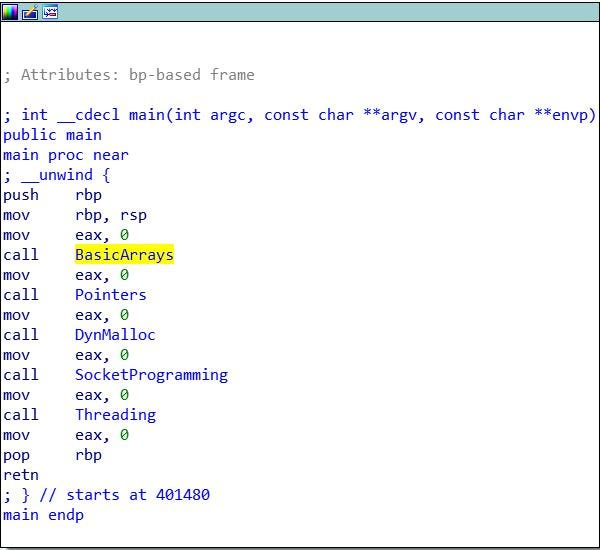Preface

Throughout this article we will be breaking down the following programming concepts and analyzing the decompiled assembly versions of each instruction:

1. Arrays
2. Pointers
3. Dynamic Memory Allocation
4. Socket Programming (Network Programming)

For the Part 1 of the BOLO: Reverse Engineering series, please click here.

Please note: While this article uses IDA Pro to disassemble the compiled code, many of the features of IDA Pro (i.e. graphing, pseudocode translation, etc.) can be found in plugins and builds for other free disassemblers such as radare2. Furthermore, while preparing for this article I took the liberty of changing some variable names in the disassembled code from IDA presets like “v20” to what they correspond to in the C code. This was done to make each portion easier to understand. Finally, please note that this C code was compiled into a 64 bit executable and disassembled with IDA Pro’s 64 bit version. This can be especially seen when calculating array sizes, as the 32 bit registers (i.e. eax) are often doubled in size and transformed into 64 bit registers (i.e rax).

Ok, Let’s begin!

While Part 1 broke down and described basic programming concepts like loops and IF statements, this article is meant to explain more advanced topics that you would have to decipher when reverse engineering.

Arrays

Let’s begin with Arrays, First, let’s take a look at the code as a whole: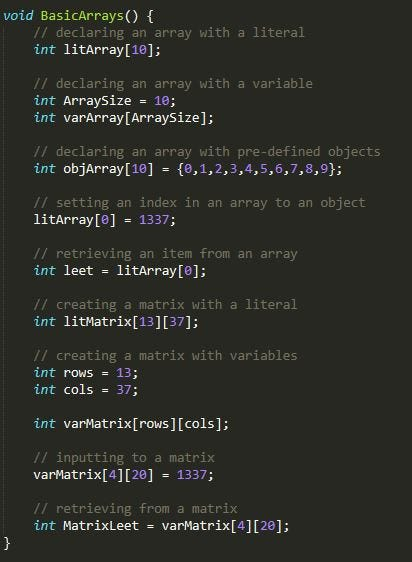Now, let’s take a look at the decompiled assembly as a whole: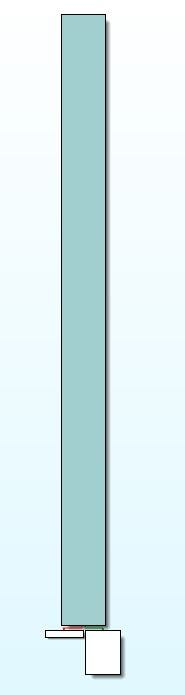As you can see, the 12 lines of code turned into quite a large block of code. But don’t be intimidated! Remember, all we’re doing here is setting up arrays!

Let’s break it down bit by bit: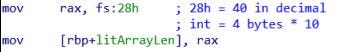When initializing an array with an integer literal, the compiler simply initializes the length through a local variable.

EDIT: The above photo labeled “Declaring an array with a literal — disassembled” is actually labeled incorrectly. While yes, when initializing an array with an integer literal the compiler does first initialize the length through a local variable, the above screenshot is actually the initialization of a stack canary. Stack Canaries are used to detect overflow attacks that may, if unmitigated, lead to execution of malicious code. During compilation the compiler allocated enough space for the only litArray element that would be used, litArray (see photo below labeled “local variables — Arrays” — as you can see, the only litArray element that was allocated for is litArray). Compiler optimization can significantly enhance the speed of applications.
Sorry for the confusion!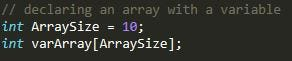Declaring an array with a variable — code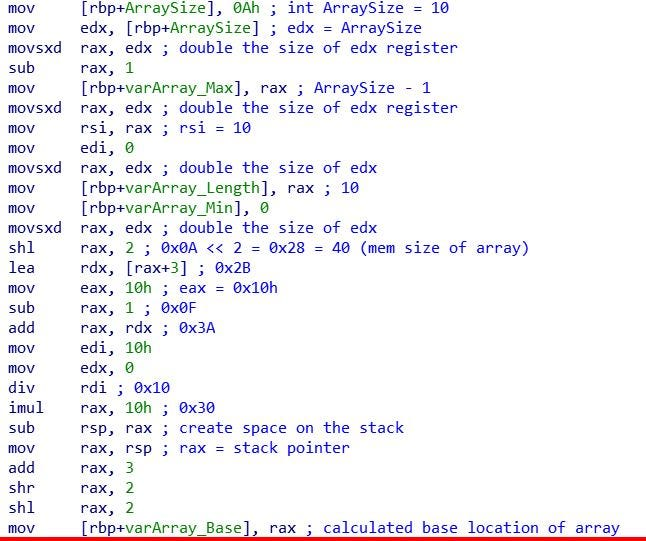Declaring an array with a variable — assembly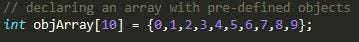declaring an array with pre-defined objects — code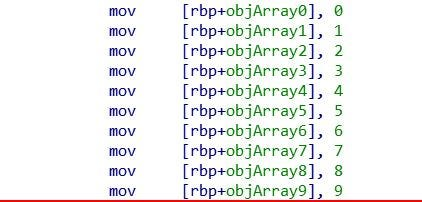When declaring an array with pre-defined index definitions the compiler simply saves each pre-defined object into its own variable which represents the index within the array (i.e. objArray4 = objArray)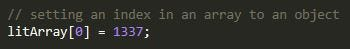initializing an array index — code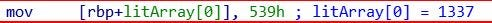initializing an array index — assembly

Much like declaring an array with pre-defined index definitions, when initializing (or setting) an index in an array, the compiler creates a new variable for said index.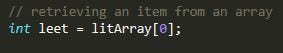retrieving an item from an array — code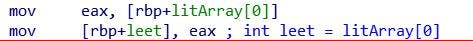retrieving an item from an array — assembly

When retrieving items from arrays, the item is taken from the index within the array and set to the desired variable.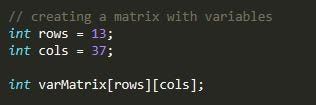creating a matrix with variables — code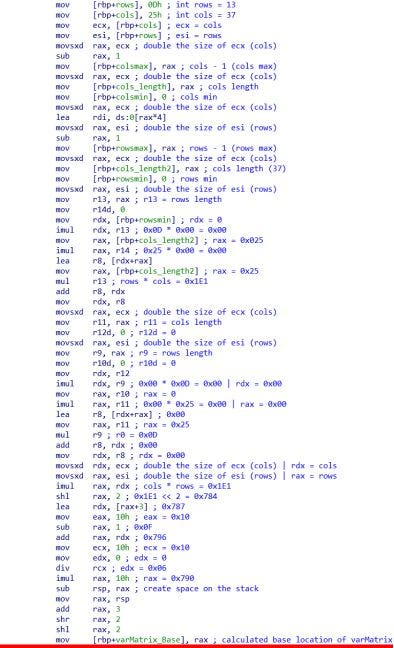When creating a matrix, first the row and column sizes are set to their row and col variables. Next, the maximum and minimum indexes for the rows and columns are calculated and used to calculate the base location / overall size of the matrix in memory.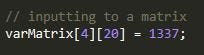inputting to a matrix — code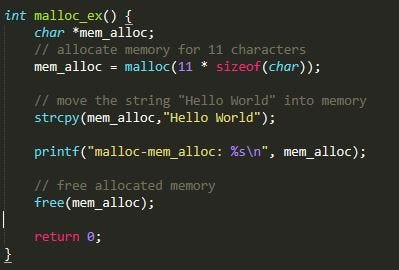Dynamic memory allocation using malloc — code

In this function we allocate 11 characters using malloc and then copy “Hello World” into the allocated memory space.

Now, let’s take a look at the assembly:

Please note: Throughout the assembly you may see ‘nop’ instructions. these instructions were specifically placed by me during the preparation stage for this article so that I could easily navigate and comment throughout the assembly code.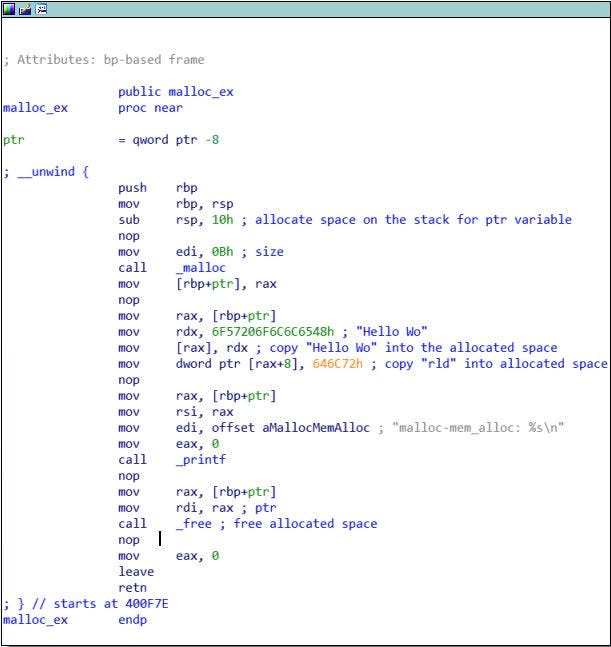dynamic memory allocation using malloc — assembly

When using malloc, first the size of the allocated memory (0x0B) is first moved into the edi register. Next, the _malloc system function is called to allocate memory. The allocated memory area is then stored in the ptr variable. Next, the “Hello World” string is broken down into “Hello Wo” and “rld” as it is copied into the allocated memory space. Finally, the newly copied “Hello World” string is printed out and the allocated memory is freed using the _free system function.

calloc — dynamic memory allocation

First, let’s take a look at the code: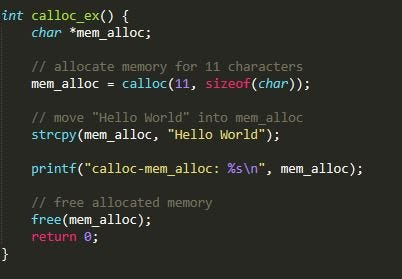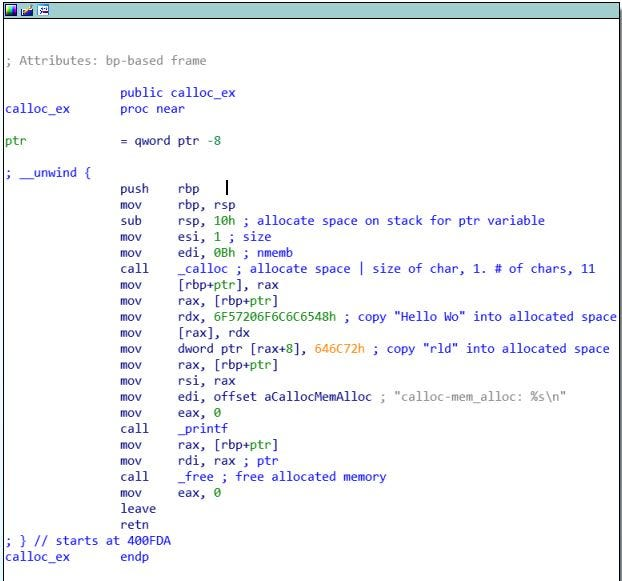dynamic memory allocation using calloc — code
Реклама

Reverse Engineering Basic Programming Concepts

Throughout the reverse engineering learning process I have found myself wanting a straightforward guide for what to look for when browsing through assembly code. While I’m a big believer in reading source code and manuals for information, I fully understand the desire to have concise, easy to comprehend, information all in one place. This “BOLO: Reverse Engineering” series is exactly that! Throughout this article series I will be showing you things to BOn the Look Out for when reverse engineering code. Ideally, this article series will make it easier for beginner reverse engineers to get a grasp on many different concepts!

Preface

Throughout this article you will see screenshots of C++ code and assembly code along with some explanation as to what you’re seeing and why things look the way they look. Furthermore, This article series will not cover the basics of assembly, it will only present patterns and decompiled code so that you can get a general understanding of what to look for / how to interpret assembly code.

1. Variable Initiation
2. Basic Output
3. Mathematical Operations
4. Functions
5. Loops (For loop / While loop)
6. Conditional Statements (IF Statement / Switch Statement)
7. User Input

please note: This tutorial was made with visual C++ in Microsoft Visual Studio 2015 (I know, outdated version). Some of the assembly code (i.e. user input with cin) will reflect that. Furthermore, I am using IDA Pro as my disassembler.

Variable Initiation

Variables are extremely important when programming, here we can see a few important variables:

1. a string
2. an int
3. a boolean
4. a char
5. a double
6. a float
7. a char array

Please note: In C++, ‘string’ is not a primitive variable but I thought it important to show you anyway.

Now, lets take a look at the assembly:

Here we can see how IDA represents space allocation for variables. As you can see, we’re allocating space for each variable before we actually initialize them.

Once space is allocated, we move the values that we want to set each variable to into the space we allocated for said variable. Although the majority of the variables are initialized here, below you will see the C++ string initiation.

As you can see, initiating a string requires a call to a built in function for initiation.

Basic Output

preface info: Throughout this section I will be talking about items pushed onto the stack and used as parameters for the printf function. The concept of function parameters will be explained in better detail later in this article.

Although this tutorial was built in visual C++, I opted to use printf rather than cout for output.

Now, let’s take a look at the assembly:

First, the string literal:

As you can see, the string literal is pushed onto the stack to be called as a parameter for the printf function.

Now, let’s take a look at one of the variable outputs:

As you can see, first the intvar variable is moved into the EAX register, which is then pushed onto the stack along with the “%i” string literal used to indicate integer output. These variables are then taken from the stack and used as parameters when calling the printf function.

Mathematical Functions

In this section, we’ll be going over the following mathematical functions:

2. Subtraction
3. Multiplication
4. Division
5. Bitwise AND
6. Bitwise OR
7. Bitwise XOR
8. Bitwise NOT
9. Bitwise Right-Shift
10. Bitwise Left-Shift

Let’s break each function down into assembly:

First, we set A to hex 0A, which represents decimal 10, and to hex 0F, which represents decimal 15.

We add by using the ‘add’ opcode:

We subtract using the ‘sub’ opcode:

We multiply using the ‘imul’ opcode:

We divide using the ‘idiv’ opcode. In this case, we also use the ‘cdq’ to double the size of EAX so that we can fit the output of the division operation.

We perform the Bitwise AND using the ‘and’ opcode:

We perform the Bitwise OR using the ‘or’ opcode:

We perform the Bitwise XOR using the ‘xor’ opcode:

We perform the Bitwise NOT using the ‘not’ opcode:

We peform the Bitwise Right-Shift using the ‘sar’ opcode:

We perform the Bitwise Left-Shift using the ‘shl’ opcode:

Function Calls

In this section, we’ll be looking at 3 different types of functions:

1. a basic void function
2. a function that returns an integer
3. a function that takes in parameters

First, let’s take a look at calling newfunc() and newfuncret() because neither of those actually take in any parameters.

If we follow the call to the newfunc() function, we can see that all it really does is print out “Hello! I’m a new function!”:

As you can see, this function does use the retn opcode but only to return back to the previous location (so that the program can continue after the function completes.) Now, let’s take a look at the newfuncret() function which generates a random integer using the C++ rand() function and then returns said integer.

First, space is allocated for the A variable. Then, the rand() function is called, which returns a value into the EAX register. Next, the EAX variable is moved into the A variable space, effectively setting A to the result of rand(). Finally, the A variable is moved into EAX so that the function can use it as a return value.

Now that we have an understanding of how to call function and what it looks like when a function returns something, let’s talk about calling functions with parameters:

First, let’s take another look at the call statement: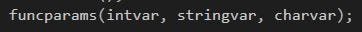Calling a Function with Parameters in C++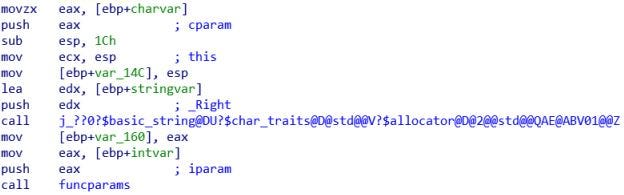Calling a Function with Parameters

Although strings in C++ require a call to a basic_string function, the concept of calling a function with parameters is the same regardless of data type. First ,you move the variable into a register, then you push the registers on the stack, then you call the function.

Let’s take a look at the function’s code:

All this function does is take in a string, an integer, and a character and print them out using printf. As you can see, first the 3 variables are allocated at the top of the function, then these variables are pushed onto the stack as parameters for the printf function. Easy Peasy.

Loops

Now that we have function calling, output, variables, and math down, let’s move on to flow control. First, we’ll start with a for loop:

Before we break down the assembly code into smaller sections, let’s take a look at the general layout. As you can see, when the for loop starts, it has 2 options; It can either go to the box on the right (green arrow) and return, or it can go to the box on the left (red arrow) and loop back to the start of the for loop.

First, we check if we’ve hit the maximum value by comparing the i variable to the max variable. If the i variable is not greater than or equal to the maxvariable, we continue down to the left and print out the i variable then add 1 to i and continue back to the start of the loop. If the i variable is, in fact, greater than or equal to max, we simply exit the for loop and return.

Now, let’s take a look at a while loop:

In this loop, all we’re doing is generating a random number between 0 and 20. If the number is greater than 10, we exit the loop and print “I’m out!” otherwise, we continue to loop.

In the assembly, the A variable is generated and set to 0 originally, then we initialize the loop by comparing A to the hex number 0A which represents decimal 10. If A is not greater than or equal to 10, we generate a new random number which is then set to A and we continue back to the comparison. If A is greater than or equal to 10, we break out of the loop, print out “I’m out” and then return.

If Statements

Next, we’ll be talking about if statements. First, let’s take a look at the code:

This function generates a random number between 0 and 20 and stores said number in the variable A. If A is greater than 15, the program will print out “greater than 15”. If A is less than 15 but greater than 10, the program will print out “less than 15, greater than 10”. This pattern will continue until A is less than 5, in which case the program will print out “less than 5”.

Now, let’s take a look at the assembly graph:

As you can see, the assembly is structured similarly to the actual code. This is because IF statements are simply “If X Then Y Else Z”. IF we look at the first set of arrows coming out of the top section, we can see a comparison between the A variable and hex 0F, which represents decimal 15. If A is greater than or equal to 15, the program will print out “greater than 15” and then return. Otherwise, the program will compare A to hex 0A which represents decimal 10. This pattern will continue until the program prints and returns.

Switch Statements

Switch statements are a lot like IF statements except in a Switch statement one variable or statement is compared to a number of ‘cases’ (or possible equivalences). Let’s take a look at our code:

In this function, we set the variable A to equal a random number between 0 and 10. Then, we compare A to a number of cases using a Switch statement. IfA is equal to any of the possible cases, the case number will be printed, and then the program will break out of the Switch statement and the function will return.

Now, let’s take a look at the assembly graph:

Unlike IF statements, switch statements do not follow the “If X Then Y Else Z” rule, instead, the program simply compares the conditional statement to the cases and only executes a case if said case is the conditional statement’s equivalent. Le’ts first take a look at the initial 2 boxes:

First, the program generates a random number and sets it to A. Then, the program initializes the switch statement by first setting a temporary variable (var_D0) to equal A, then ensuring that var_D0 meets at least one of the possible cases. If var_D0 needs to default, the program follows the green arrow down to the final return section (see below). Otherwise, the program initiates a switch jump to the equivalent case’s section:

In the case that var_D0 (A) is equal to 5, the code will jump to the above case section, print out “5” and then jump to the return section.

User Input

In this section, we’ll cover user input using the C++ cin function. First, let’s look at the code:

In this function, we simply take in a string to the variable sentence using the C++ cin function and then we print out sentence through a printf statement.

Le’ts break this down into assembly. First, the C++ cin part: